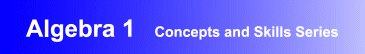Award-Winning

# Algebra 1 Interactive Tutorial Software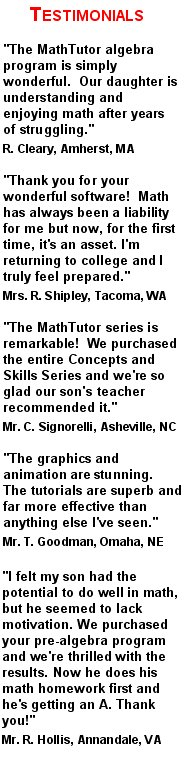DESCRIPTION
ALGEBRA 1 is the ideal

## interactive learning and tutorial program for first year algebra.

It is the top rated self study algebra software program, and has helped students at all levels of ability reach their potential.
Schools seeking

## curriculum-based adaptive instructional software for algebra 1

will find the program to be perfectly suited to their needs.
Parents wanting homeschool algebra 1 self-paced learning software will find the program to be ideal.
Dynamic, engaging lessons use graphics and animation to help students learn the concepts of elementary algebra, master problem-solving, and gain the confidence so important to future math success.
Educators agree that the program is a breakthrough in

## algebra tutorial software

. Carefully crafted lessons provide a unique learning experience and help students gain a strong understanding of the concepts of algebra. In addition, students receive personalized on-demand coaching and hints as they learn. Dr. Jonathan Friedman, one of the program's authors says "ALGEBRA 1 lets students work at their own pace and truly master each concept. As a result, they feel confident and truly prepared before moving on to the next unit of learning."
ALGEBRA 1 has been acclaimed for its educational excellence, design, and flexibility. It can be used for self-study and to supplement classroom instruction in high school and middle school math classes. The program also provides diagnostic and assessment features which create individualized study plans for students.
Designed by math educators and research based, the program provides interactive algebra 1 lessons suitable for students at all levels of ability. The program is also effective when used as software for developmental and remedial math teaching.
• Award-winning, curriculum-based algebra 1 courseware developed by math educators
• Provides dynamic, interactive algebra 1 lessons that are ideal for self-study, and as a supplement to classroom instruction
• Demonstrated success in boosting students' math skills and comprehension, and proficiency in problem solving
• Network versions contain student record-keeping, grading, and performance analysis features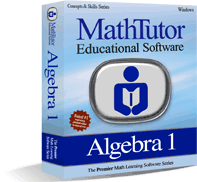TOPICS COVERED
Review of Arithmetic
Fundamental Operations
• Properties of Addition
• Properties of Multiplication
• The Distributive Property
Equations
• Like Terms
• Simplifying Expressions
• Properties of Equations
• Solving Linear Equations with One Operation
• Solving Linear Equations with Two Operations
• Solving More Complex Linear Equations
• Solving Literal Equations and Formulas
Solving Word Problems
Exponents and Powers
Polynomials
• Simplifying Polynomials
• Adding and Subtracting Polynomials
• Multiplying Polynomials
• Multiplying Polynomials Using FOIL
• Dividing Polynomials
• Evaluating Functions
Factoring
• Prime Factorization
• Removing the Greatest Common Factor
• Trinomials of the Form x2+bx+c
• Trinomials of the Form ax2+bx+c
• Perfect Square Trinomials
• Difference of Two Squares
• Using Grouping to Factor Trinomials
• Using Factoring to Solve Equations
Graphs and Linear Equations
Rational Expressions
• Defining Rational Expressions
• Multiplying and Dividing Rational Expressions
• Finding the LCM and GCD
• Adding and Subtracting Rational Expressions
• Simplifying Complex Fractions
• Rational Expressions: Solving Equations
Systems of Equations
• Solving Systems by Graphing
• Solving Systems by Addition
• Solving Systems by Substitution
• Word Problems
Inequalities
Radicals
• Simplifying Radicals
• Simplifying Radical Expressions
• Adding and Subtracting Radicals
• Rationalizing the Denominator
Quadratic Equations
• Solving Quadratic Equations
• Completing the Square
• The Quadratic Formula
• Graphing Quadratics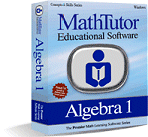Order Now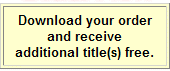TOPICS COVERED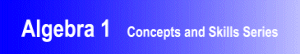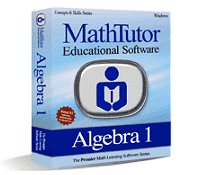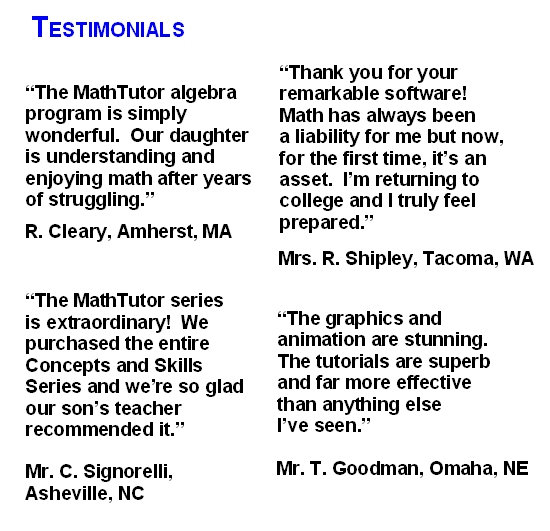TOPICS COVERED